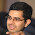## Monday, 13 August 2012

### A 10 digit number

Consider a 10-digit number A written as a_0a_1a_2...a_9. Now, suppose a_0 is the number of times the digit '0' appears in A; a_1 is the number of times '1' appears in A, and so on... a_9 is the number of times '9' appears. What is A?

#### 1 comment:

1.6210001000

(1)--> SUM(a_i) = 10
(2)--> if a_0 = n, then there must be exactly 9-n non-zero digits in the number which add up to 10-n.

Using (1), (2) and the hypothesis of the problem, it is easy to deduce that a_0 can only be 6. The rest of the digits can now be easily found out.